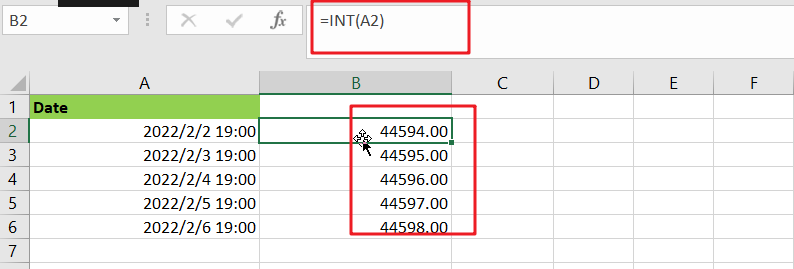# Extract date from multiple cells containing both date and time

As if you have got a cell containing date along with the time in the same cell and you want to extract the date into a separate cell, then for one or two cells, you can extract the date manually from the cell, but if you have got multiple cells in excel containing both date and time in the same cell then doing it manually would be very tough you would get tired of it and would never complete your work on time.

But don’t be worry about it because after carefully reading this article, extracting date from the multiple cells containing both date and time would become a piece of cake for you, as it is done in the following screenshot, So let’s dive into it:

## General Formula:

The Following formula would help you out for extracting date from the multiple cells containing both date and time to another cell; in the above example, the formula is shown in the cell B2:

`=INT(date_value)`

## Let’s See How This Formula Works:

You would definitely wonder that how excel efficiently and precisely extracts date from the multiple cells containing both date and time? Then here is the answer that the excel handles it by several numbers and fractional values, which means that Excel uses a pattern in which it denotes the dates as the several numbers and time to the fractional values.

For instance, suppose that both date and time  `Feb 2, 2022, 19:00 PM` are in the same cell, as now in Ms excel this date and time are represented in terms of numbers as “`44594.7916666667`, where the “`44594`” is the date portion, and the fractional part “`.``7916666667`” is the time portion.

So if you want to extract date from the cells that include both the date and time, you can use the INT function to extract only the date part. The INT function returns only the decimal portion from each cell by excluding the fractional part, and you would get the date from each cell efficiently and precisely.

As by the aid of an example, you would understand it more clearly, so let suppose in A2 there are both date and time which is Feb 2, 2022, 19:00 PM, which is equivalent to the number 44594.7916666667 in MS Excel, so when you would use the INT function which is given below then it would return the date portion (44594):

`=INT(A2)`Obviously, the fractional part or the time portion is discarded to get the date portion, but for that, be sure you are using a date format that does not include a time. Otherwise, according to the above example, you will see the time displayed as 19:00 PM instead of the date portion.

### Related Functions

• Excel INT function
The Excel INT function returns the integer portion of a given number. And it will rounds a given number down to the nearest integer.The syntax of the INT function is as below:= INT (number)…